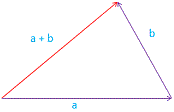#### Vector Calculator

Calculator that allows you to calculate the sum of two dimensional vectors with the entered values (vector coordinates).

 Vector a: ; Vector b: .

In algebra vector addition takes into account for direction and magnitude of the vector. The magnitude is used to describe the length of physical quantities which have both magnitude and direction associated with them.Lets consider two vectors a and b. vector a and b may be velocity vectors / displacement vectors / electric field vectors / any type of vectors.

We draw vector a then draw vector b from the tip of vector a as shown in picture. The sum of vectors is called the resultant.

This resultant vector r we can draw from the beginning of vendor a to end of vector b, we can write as r = a + b.

The important to remember that vector addition is commutative so that resultants are exactly the same. That means the order in which vectors are added does not affect the resultant.

In addition we can begin by drawing vector b at the end of vector b we will draw vector a. The resultant vector we can draw by starting at the beginning of b and finishing at the end of vector , we can write as r = b + a.